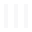NAV# Welcome to the API docs for RIDDLE!

Check version

``````import riddle
``````
``````riddle.hello()
``````
``````  > Hello, World
``````
``````  > My name is RIDDLE 2.0.0

``````

RIDDLE (Race and ethnicity Imputation from Disease history with Deep LEarning) is an open-source Python2 library for using deep learning to impute race and ethnicity information in anonymized electronic medical records (EMRs). RIDDLE provides the ability to (1) build models for estimating race and ethnicity from clinical features, and (2) interpret trained models to describe how specific features contribute to predictions. The RIDDLE library implements the methods introduced in “RIDDLE: Race and ethnicity Imputation from Disease history with Deep LEarning” (PLOS Computational Biology, 2018).

Compared to alternative methods (e.g., scikit-learn/Python, glm/R), RIDDLE is designed to handle large and high-dimensional datasets in a performant fashion. RIDDLE trains models efficiently by using a parallelized TensorFlow-under-Keras backend, and avoids memory overflow by preprocessing data in conjunction with batch-wise training.

RIDDLE uses Keras to specify and train the underlying deep neural networks, and DeepLIFT to compute feature-to-class contribution scores. The default architecture is a deep multi-layer perceptron (deep MLP) that takes binary-encoded features and targets. However, you can specify any neural network architecture (e.g., LSTM, CNN) and data format by writing your own `model_module` files (see Configuration)!

# Installation

Shell commands:

``````# Install HDF5 (only non-pip dependency)
``````
``````apt-get install libhdf5-serial-dev

``````
``````# Option 1) Clone from GitHub
``````
``````git clone --recursive git://github.com/jisungk/riddle.git
``````
``````cd riddle
``````
``````pip install -r requirements.txt
``````
``````apt-get install libhdf5-serial-dev

``````
``````# Option 2) Install using pip
``````
``````pip install git+https://github.com/jisungk/riddle
``````
``````pip install git+https://github.com/kundajelab/deeplift
``````
``````
``````

### Install the following libraries/software:

• RIDDLE (`riddle`, clone from GitHub)
• DeepLIFT (`deeplift`, submodule in RIDDLE repository)
• Keras (`keras`)
• TensorFlow (`tensorflow`)
• scikit-learn (`sklearn`)
• NumPy (`numpy`)
• SciPy (`scipy`)
• Matplotlib (`matplotlib`)
• h5py (`h5py`)

• HDF5

### Configuration

• Configure `feature_importance.py` to point to the correct `deeplift` directory.
• Modify `parameter_search.py`, `riddle.py`, `feature_importance.py` as needed (e.g., datapath FLAGS).
• If desired, write your own architecture class which inherits from the `models/model.Model` class. Update the above scripts accordingly.

# High-level API

Quickstart commands

``````# run in repository directory
``````
``````python parameter_search.py
``````
``````python riddle.py
``````
``````python interpret_riddle.py
``````

Template script for a toy pipeline

``````import numpy as np
``````
``````from sklearn.metrics import accuracy_score

``````
``````from riddle import emr, models

``````
``````# get data
``````
``````x, y = emr.get_data(...)
``````
``````x_train, y_train, x_val, y_val, x_test, y_test = emr.get_k_fold_partition(x, y, ...)

``````
``````# specify model
``````
``````model = MLP(...)

``````
``````# train and evaluate model
``````
``````model.train(x_train, y_train, x_val, y_val)

``````
``````y_probas = model.predict_proba(x_test)
``````
``````y_pred = np.argmax(y_probas, axis=1)
``````
``````print('accuracy: {:.4f}'.format(accuracy_score(y_test, y_pred)))

``````

### Modules

Module Description
`riddle/emr.py` Reads in data files & preprocesses the data.
`riddle/feature_importance.py` Computes & summarizes DeepLIFT feature contribution scores
`riddle/roc.py` Plots ROC curves and computes ROC AUC scores
`riddle/tuning.py` Implements parameter tuning functions
`riddle/models/model.py` Base Model class for defining model architectures
`riddle/models/mlp.py` MLP architecture (used in the PLOS CB paper)

### Scripts

Script Description
`parameter_tuning.py` Runs parameter tuning
`riddle.py` Runs experiments (model training and evaluation) using k-fold cross-validation
`interpret_riddle.py` Runs a pipeline to compute DeepLIFT scores

# Authors

Ji-Sung Kim
Princeton University
hello (at) jisungkim.com (technical inquiries)

Xin Gao, Associate Professor
King Abdullah University of Science and Technology

Andrey Rzhetsky, Edna K. Papazian Professor
University of Chicago
andrey.rzhetsky (at) uchicago.edu (research inquiries)